### Practical Geometry-Solutions 14.6

CBSE Class –VI Mathematics
NCERT Solutions
Chaper 14 Practical Geometry (Ex. 14.6)

Question 1. Draw $\mathrm{\angle }$POA of measure ${75}^{\circ }$ and find its line of symmetry.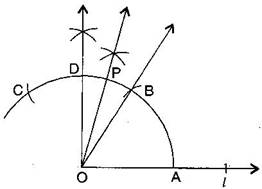(a) Draw a line $l$ and mark a point O on it.
(b) Place the pointer of the compasses at O and draw an arc of any radius which intersects the line $l$ at A.
(c) Taking the same radius, with centre A, cut the previous arc at B.
(d) Join OB, then $\mathrm{\angle }$BOA = ${60}^{\circ }.$
(e) Taking the same radius, with centre B, cut the previous arc at C.
(f) Draw bisector of $\mathrm{\angle }$BOC. The angle is of ${90}^{\circ }.$ Mark it at D. Thus, $\mathrm{\angle }$DOA = ${90}^{\circ }$
(g) Draw $\overline{\text{OP}}$ as bisector of $\mathrm{\angle }$DOB.
Thus, $\mathrm{\angle }$POA = ${75}^{\circ }$
Question 2. Draw an angle of measure ${147}^{\circ }$ and construct its bisector.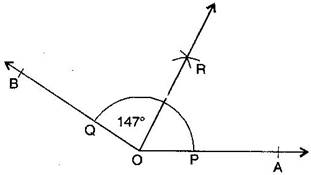(a) Draw a ray $\stackrel{↔}{\text{OA}}.$
(b) With the help of protractor, construct $\mathrm{\angle }$AOB = ${147}^{\circ }.$
(c) Taking centre O and any convenient radius, draw an arc which intersects the arms $\overline{\text{OA}}$ and $\overline{\text{OB}}$ at P and Q respectively.
(d) Taking P as centre and radius more than half of PQ, draw an arc.
(e) Taking Q as the centre and with the same radius, draw another arc which intersects the previous at R.
(f) Join OR and produce it.
Thus, $\overline{\text{OR}}$ is the required bisector of $\mathrm{\angle }$AOB.
Question 3. Draw a right angle and construct its bisector.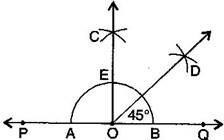(a) Draw a line PQ and take a point O on it.
(b) Taking O as the centre and convenient radius, draw an arc which intersects PQ at A and B.
(c) Taking A and B as centres and radius more than half of AB, draw two arcs which intersect each other at C.
(d) Join OC. Thus, $\mathrm{\angle }$COQ is the required right angle.
(e) Taking B and E as centre and radius more than half of BE, draw two arcs which intersect each other at the point D.
(f) Join OD. Thus, $\overline{\text{OD}}$ is the required bisector of $\mathrm{\angle }$COQ.
Question 4. Draw an angle of measure ${153}^{\circ }$ and divide it into four equal parts.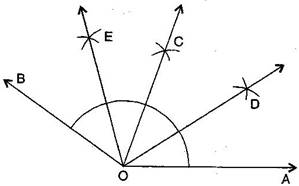(a) Draw a ray $\stackrel{↔}{\text{OA}}.$
(b) At O, with the help of a protractor, construct $\mathrm{\angle }$AOB = ${153}^{\circ }.$
(c) Draw $\overline{\text{OC}}$ as the bisector of $\mathrm{\angle }$AOB.
(d) Again, draw $\overline{\text{OD}}$ as bisector of $\mathrm{\angle }$AOC.
(e) Again, draw $\overline{\text{OE}}$ as bisector of $\mathrm{\angle }$BOC.
(f) Thus, $\overline{\text{OC}}$$\overline{\text{OD}}$ and $\overline{\text{OE}}$ divide $\mathrm{\angle }$AOB in four equal arts.
Question 5. Construct with ruler and compasses, angles of following measures:
(a) ${60}^{\circ }$
(b) ${30}^{\circ }$
(c) ${90}^{\circ }$
(d) ${120}^{\circ }$
(e) ${45}^{\circ }$
(f) ${135}^{\circ }$
Steps of construction: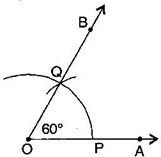(a) ${60}^{\circ }$
(i) Draw a ray $\stackrel{↔}{\text{OA}}.$
(ii) Taking O as the centre and convenient radius, mark an arc, which intersects $\stackrel{↔}{\text{OA}}$ at P.
(iii) Taking P as the centre and the same radius, cut the previous arc at Q.
(iv) Join OQ and extend to B
Thus, $\mathrm{\angle }$BOA is required angle of ${60}^{\circ }.$
(b) ${30}^{\circ }$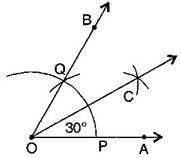(i) Draw a ray $\stackrel{↔}{\text{OA}}.$
(ii) Taking O as the centre and convenient radius, mark an arc, which intersects $\stackrel{↔}{\text{OA}}$ at P.
(iii) Taking P as the centre and the same radius, cut the previous arc at Q.
(iv) Join OQ. Thus, $\mathrm{\angle }$BOA is the required angle of ${60}^{\circ }.$
(v) Put the pointer on P and mark an arc.
(vi) Put the pointer on Q and with the same radius, cut the previous arc at C.
Thus, $\mathrm{\angle }$COA is required angle of ${30}^{\circ }.$
(c) ${90}^{\circ }$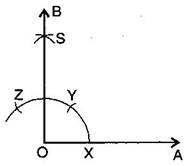(i) Draw a ray $\stackrel{↔}{\text{OA}}.$
(ii) Taking O as the centre and convenient radius, mark an arc, which intersects $\stackrel{↔}{\text{OA}}$ at X.
(iii) Taking X as the centre and the same radius, cut the previous arc at Y.
(iv) Taking Y as the centre and the same radius, draw another arc intersecting the same arc at Z.
(v) Taking Y and Z as centres and the same radius, draw two arcs intersecting each other at S.
(vi) Join OS and produce it to form a ray OB.
Thus, $\mathrm{\angle }$BOA is required angle of ${90}^{\circ }.$
(d) ${120}^{\circ }$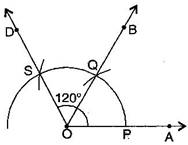(i) Draw a ray $\stackrel{↔}{\text{OA}}.$
(ii) Taking O as the centre and convenient radius, mark an arc, which intersects $\stackrel{↔}{\text{OA}}$ at P.
(iii) Taking P as the centre and the same radius, cut the previous arc at Q.
(iv) Taking Q as the centre and the same radius cut the arc at S.
(v) Join OS.
Thus, $\mathrm{\angle }$AOD is required angle of ${120}^{\circ }.$
(e) ${45}^{o}$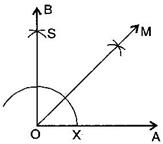points y and z are not marked in the figure
(i) Draw a ray $\stackrel{\to }{OA}$
(ii) Taking O as the centre and convenient radius, mark an arc, which intersects $\stackrel{\to }{OA}$ at X.
(iii) Taking X as the centre and the same radius, cut the previous arc at Y.
(iv) Taking Y as the centre and the same radius, draw another arc intersecting the same arc at Z.
(v) Taking Y and Z as centres and the same radius, draw two arcs intersecting each other at S.
(vi) Join OS and produce it to form a ray OB. Thus, $\mathrm{\angle }$BOA is required angle of ${90}^{o}$
(vii) Draw the bisector of $\mathrm{\angle }$BOA.
Thus, $\mathrm{\angle }$MOA is required angle of ${45}^{o}$
(f) ${135}^{o}$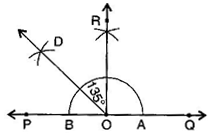the angle 135 degrees should be shown in between QOD
(i) Draw a line PQ and take a point O on it.
(ii) Taking O as the centre and convenient radius, mark an arc, which intersects PQ at A and B.
(iii) Taking A and B as centres and radius more than half of AB, draw two arcs intersecting each other at R.
(iv) Join OR. Thus, $\mathrm{\angle }$QOR = $\mathrm{\angle }$POR = ${90}^{o}$
(v) Draw $\stackrel{\to }{OD}$ the bisector of $\mathrm{\angle }$POR.
thus, $\mathrm{\angle }$QOD is the required angle of ${135}^{o}$
Question 6.Draw an angle of measure ${45}^{\circ }$ and bisect it.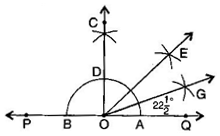(a)Draw a line PQ and take a point O on it.
(b)Taking O as the centre and a convenient radius, draw an arc which intersects PQ at two points A and B.
(c)Taking A and B as centres and radius more than half of AB, draw two arcs which intersect each other at C.
(d)Join OC. Then $\mathrm{\angle }$COQ is an angle of ${90}^{o}$
(e)Draw $\stackrel{\to }{OE}$ as the bisector of $\mathrm{\angle }$COQ. Thus, $\mathrm{\angle }$QOE = ${45}^{o}$
(f)Again draw $\stackrel{\to }{OG}$ as the bisector of $\mathrm{\angle }$QOE.
Thus, $\mathrm{\angle }$QOG = $\mathrm{\angle }$EOG = $22\frac{1}{2}{\phantom{\rule{thinmathspace}{0ex}}}^{o}$
Question 7.Draw an angle of measure ${135}^{\circ }$ and bisect it.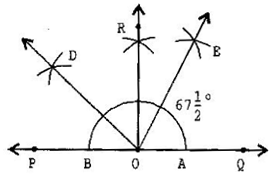(a) Draw a line PQ and take a point O on it.
(b) Taking O as a centre and convenient radius, mark an arc, which intersects PQ at A and B.
(c) Taking A and B as centres and radius more than half of AB, draw two arcs intersecting each other at R.
(d) Join OR. Thus, $\mathrm{\angle }$QOR = $\mathrm{\angle }$POQ = ${90}^{o}$
(e) Draw $\stackrel{\to }{OD}$ the bisector of $\mathrm{\angle }$POR. Thus, $\mathrm{\angle }$QOD is the required angle of ${135}^{o}$
(f) Now, draw $\stackrel{\to }{OE}$ as the bisector of $\mathrm{\angle }$QOD.
Thus, $\mathrm{\angle }$QOE = $\mathrm{\angle }$DOE = $67\frac{1}{2}{\phantom{\rule{thinmathspace}{0ex}}}^{o}$
Question 8.Draw an angle of ${70}^{\circ }.$ Make a copy of it using only a straight edge and compasses.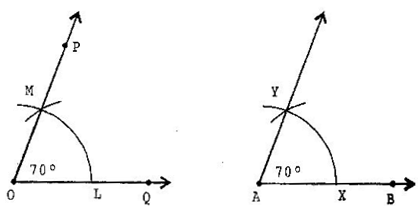(a) Draw an angle ${70}^{o}$ with protractor, i.e., $\mathrm{\angle }POQ={70}^{o}$
(b) Draw a ray $\stackrel{\to }{AB}$
(c) Place the compasses at O and draw an arc to cut the rays of $\mathrm{\angle }$POQ at L and M.
(d) Use the same compasses, setting to draw an arc with A as the centre, cutting AB at X.
(e) Set your compasses setting to the length LM with the same radius.
(f) Place the compasses pointer at X and draw the arc to cut the arc drawn earlier at Y.
(g) Join AY.
Thus, $\mathrm{\angle }YAX={70}^{o}$
Question 9.Draw an angle of ${40}^{\circ }.$ Copy its supplementary angle.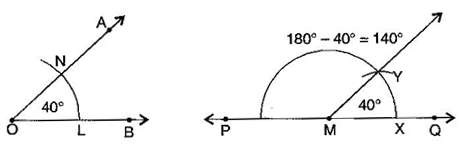(a) Draw an angle of ${40}^{o}$ with the help of protractor, naming $\mathrm{\angle }$AOB.
(d) Place the compasses at O and draw an arc to cut the rays of $\mathrm{\angle }$AOB at L and N.
Thus, $\mathrm{\angle }$QMY = ${40}^{o}$ and $\mathrm{\angle }$PMY =100 degrees is supplementary of it.